# Example implementations that use hypnettorch

Let’s dive into some example implementations that make use of the functionalities provided by the package hypnettorch. You can explore the corresponding source code to see how to efficiently make use of all the functionalities that hypnettorch offers.

## Continual learning with hypernetworks

In continual learning (CL), a series of tasks (represented as datasets)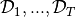is learned sequentially, where only one dataset at a time is available and at the end of training performance on all tasks should be high.

An approach based on hypernets for tackling this problem was introduced by von Oswald, Henning, Sacramento et al.. The official implementation can be found here. Goal of this example is it to demonstrate how hypnettorch can be used to implement such CL approach. Therefore, we provide a simple and light implementation that showcases many functionalities inherent to the package, but do not focus on being able to reproduce the variety of experiments explored in the original paper.

For the sake of simplicity, we only focus on the simplest CL scenario, called task-incremental CL or CL1 (note, that the original paper proposes three ways of tackling more complex CL scenarios, one of which has been further studied in this paper). Predictions according to a taskare made by inputting the corresponding task embedding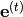into the hypernetwork in order to obtain the main network’s weights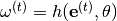, which in turn can be used for processing inputs via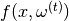. Forgetting is prevented by adding a simple regularizer to the loss while learning task:

(1)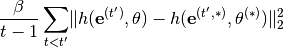where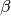is a regularization constant,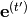are the task-embeddings,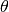are the hypernets’ parameters and parameters denoted by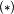are checkpointed from before starting to learn task. Simply speaking, the regularizer aims to prevent that the hypernetwork output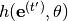for a previous task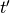changes compared to what was outputted before we started to learn task.

Note

The original paper uses a lookahead in the regularizer which showed marginal performance improvements. Follow-up work (e.g., here and here) discarded this lookahead for computational convenience. We ignore it as well!

### Usage instructions

The script hypnettorch.examples.hypercl.run showcases how a versatile simulation can be build with relatively little coding effort. You can explore the basic functionality of the script via

$python run.py --help  Note The default arguments have not been hyperparameter-searched and may thus not reflect best possible performance. By default, the script will run a SplitMNIST simulation (argument --cl_exp) $ python run.py


The default network (argument --net_type) is a 2-hidden-layer MLP and the corresponding hypernetwork has been chosen to have roughly the same number of parameters (compression ratio is approx. 1).

Via the argument --hnet_reg_batch_size you can choose up to how many task should be used for the regularization in Eq. (1) (rather than always evaluating the sum over all previous tasks). This ensures that the computational budget of the regularization doesn’t grow with the number of tasks. For instance, if at every iteration a single random (previous) task should be selected for regularization, just use

\$ python run.py --hnet_reg_batch_size=1


You can also run other CL experiments, such as PermutedMNIST (e.g., via arguments --cl_exp=permmnist --num_classes_per_task=10 --num_tasks=10) or SplitCIFAR-10/100 (e.g., via arguments --cl_exp=splitcifar --num_classes_per_task=10 --num_tasks=6 --net_type=resnet). Keep in mind, that with a change in dataset or main network, model sizes change and thus another hypernetwork should be chosen if a certain compression ratio should be accomplished.

### Learning from the example

Goal of this example is it to get familiar with the capabilities of the package hypnettorch. This can best be accomplished by reading through the source code, starting with the main function hypnettorch.examples.hypercl.run.run().

1. The script makes use of module hypnettorch.utils.cli_args for defining command-line arguments. With a few lines of code, a large variety of arguments are created to, for instance, flexibly determine the architecture of the main- and hypernetwork.

2. Using those predefined arguments allows to quickly instantiate the corresponding networks by using functions of module hypnettorch.utils.sim_utils.

3. Continual learning datasets are generated with the help of specialized data handlers, e.g., hypnettorch.data.special.split_mnist.get_split_mnist_handlers().

4. Hypernet regularization (Eq. (1)) is easily realized via the helper functions in module hypnettorch.utils.hnet_regularizer.

There are many other utilities that might be useful, but that are not incorporated in the example for the sake of simplicity. For instance:

• The module hypnettorch.utils.torch_ckpts can be used to easily save and load networks.

• The script can be emebedded into the hyperparameter-search framework of subpackage hpsearch to easily scan for hyperparameters that yield good performance.

More sophisticated examples can also be explored in the PR-CL repository (note, the interface used in this repository is almost identical to hypnettorch’s interface, except that the package wasn’t called hypnettorch back then yet).

#### Script to run CL experiments with hypernetworks

This script showcases the usage of hypnettorch by demonstrating how to use the pacakge for writing a continual learning simulation that utilizes hypernetworks. See here for details on the approach and usage instructions.

hypnettorch.examples.hypercl.run.evaluate(task_id, data, mnet, hnet, device, config, logger, writer, train_iter)[source]

Evaluate the network.

Evaluate the performance of the network on a single task on the validation set during training.

Parameters:

(....)

See docstring of function train().

train_iter (int): The current training iteration.

Parameters:
• config (argparse.Namespace) – Command-line arguments.

• logger (logging.Logger) – Logger object.

• writer (tensorboardX.SummaryWriter) – Tensorboard logger.

Returns:

A list of data handlers hypnettorch.data.dataset.Dataset.

Return type:

(list)

hypnettorch.examples.hypercl.run.run()[source]

Run the script.

1. Define and parse command-line arguments

2. Setup environment

4. Instantiate models

5. Run training for each task

hypnettorch.examples.hypercl.run.test(dhandlers, mnet, hnet, device, config, logger, writer)[source]

Evaluate the network.

Evaluate the performance of the network on a single task on the validation set during training.

Parameters:
• (....) – See docstring of function train().

• dhandlers (list) – Datasets of tasks that should be tested. We assume that the index of the dataset corresponds to the index of the task embedding used as input to the hypernet.

hypnettorch.examples.hypercl.run.train(task_id, data, mnet, hnet, device, config, logger, writer)[source]

Train the network using the task-specific loss plus a regularizer that should mitigate catastrophic forgetting.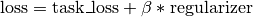Parameters: